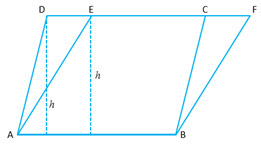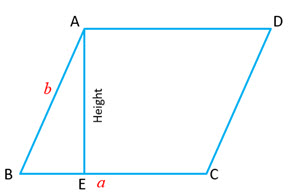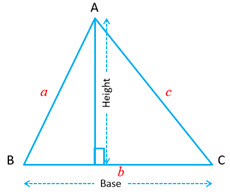Area Axioms

Area Axiom: Every polygonal region has an area and is measured in square units.

Congruent Area Axiom: If$\triangle ABC$ and$\triangle DEF$ are two congruent triangles, then$ar(\triangle ABC) = ar(\triangle DEF)$. ie. two congruent regions have equal area.

Rectangle Area Axiom: If$ABCD$ is a rectangular  region such that$AB = a$ units and$BC = b$ units, then$ar(ABCD) = ab$ square units.

Parallelograms on the same base and between the same parallelsTheorem 1: A diagonal of a parallelogram divides it into two triangles of same area

In this case$ar (\triangle ABC) = ar (\triangle ADC)$

Also$ar (\triangle ABD) = ar (\triangle BCD)$

Theorem 2: Parallelograms on the same base and between the same parallels are equal  in area.$ar (ABCD) = AB \times h$$ar (ABFE) = AB \times h$$\therefore ar (ABCD) = ar (ABFE)$

Theorem 3: Area of a parallelogram is the product of its base and the corresponding altitude.Let the two adjacent sides of the parallelogram be$a$ and$b$.  Area$= Base \times Height$

Triangle Area Axiom

Theorem 4: The area of a triangle is half the product of any  of its sides and the corresponding altitude.$a, b, c$ denotes the sides of the Triangle. Then:$\displaystyle \text{Area } = \frac{1}{2} \times Base \times Height = \frac{1}{2} bh$

Area$= \sqrt{s(s-a)(s-b)(s-c)}$. This is known as Heron’s Theorem.

Theorem 5: If a triangle and parallelogram are on the same base and between the same parallels, the area of the triangle is equal to the half of the parallelogram.$ar (ABCD) = AB \times h$$\displaystyle ar ( \triangle ABE) = \frac{1}{2} \times AB \times h$$ar ( \triangle ABE) = ar (ABCD)$

Trapezium Area Axiom

Theorem 6: The area of a trapezium is half the product of its heights and the sum of parallel sides.A trapezium is a quadrilateral two of whose sides are parallel. A trapezium whose non-parallel sides are equal is known as an isosceles trapezium.

Let$a$ and$b$ be the parallel sides and$h$ be the distance between the parallel sides.$\displaystyle \text{Then Area } = \frac{1}{2} (a+b) \times h$

Triangles on the same base and between the same parallels

Theorem 7: Triangles on the same base and the same parallels have the same area.$\displaystyle ar ( \triangle ABD) = \frac{1}{2} \times AB \times h$$\displaystyle ar ( \triangle ABC) = \frac{1}{2} \times AB \times h$$\displaystyle \therefore ar ( \triangle ABD) = ar ( \triangle ABC)$

Theorem 8: Triangles having equal areas and having one side of one of the triangles equal to one side of the other triangle, have their corresponding altitudes the same.

Theorem 9: Two triangles having the same bases (or equal bases) and equal area lie between the same parallels.